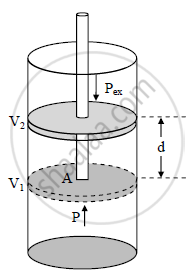# Derive the equation : W = - PextAV - Chemistry

Derive the equation : W = - PextAV

#### Solution1. The work W, that is done due to the expansion or compression of a gas against an external opposing pressure P is called pressure-volume work.
2. Consider a certain amount of gas at pressure P, volume V1 and temperature T enclosed in a cylinder fitted with frictionless, rigid, movable piston of area A as shown in the figure.
3. As the gas expands, it pushes the piston upward through a distance d against an external opposing force f. The work done by the gas during the process is given by,
W = opposing force × distance = -f.d ....(1)
4. The negative sign signifies that when the piston moves against an opposing force, the internal energy of the system doing the work will decrease. The force opposing the expansion is the constant external pressure, pex multiplied by the area A of the piston.
f = pex . A ....(2)
From equations (1) and (2),
W = -pex × A × d
5. The product A.d is the volume of the cylinder covered by the piston during its motion that is the change in volume during expansion. Thus
A × d = ΔV = V2 - V1
where, V2 is the final volume of the gas.
W = -pex ⋅ ΔV = -pex (V2 - V1)

Concept: Chemical Thermodynamics and Energetic - Work, Heat, Energy, Extensive and Intensive Properties
Is there an error in this question or solution?